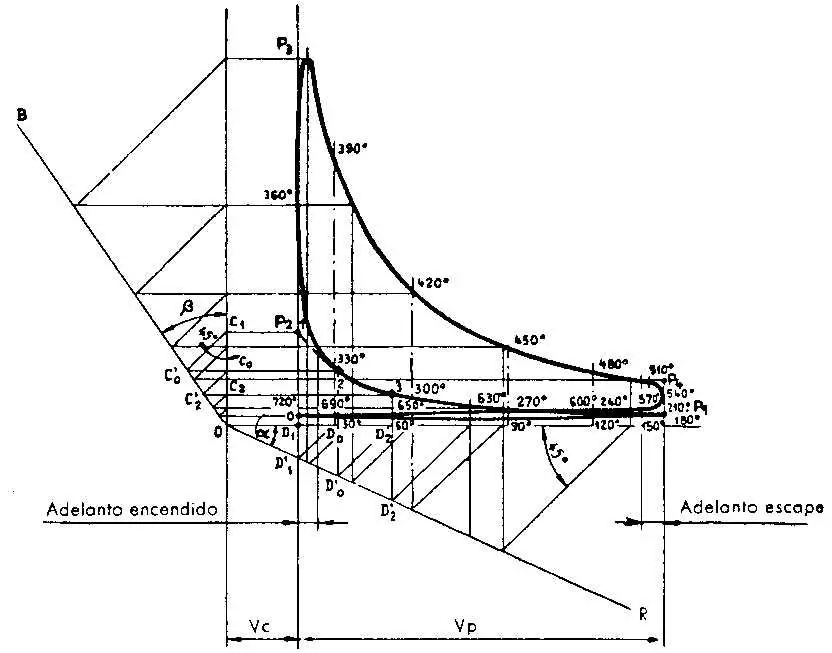# Indicated CycleTo determine the value of the forces acting on the motor organs it is important to know the cycle well. In addition, it will allow us to measure the engine parts during the development of the project.

This can be traced by similarity with similar motor cycles with the one under study. This information is also obtained in a simple and fast way, with a sufficient approximation, using the following method, which we set out as an example and recommend as applicable in the case of 4-stroke and spark-ignition engines.

Knowing the diameter, the stroke and the compression ratio p, we calculate the volume of the compression chamber Vc, or that of the unit displacement, Vp, whose values ​​Vc and Vc + Vp take as abscissa a Cartesian diagram. In correspondence with these abscissa we carry as ordered the respective pressures registered at the end of each of the phases. In practice, for the Otto cycle its values ​​are set, with discrete approximation, as follows:

p1 = pressure at the end of aspiration = between 0.9 and 1 Kg / cm 2 ;
p2 = pressure at the end of compression = p1 p ^ nc;
p3 = maximum pressure at the end of combustion = 7 p - 2;
p4 = expansion stop pressure = p3 / p ^ ne;

where:

nc = exponent of compression polytropics;
ne = exponent of the expansion policy.

Both polytropics are drawn according to the construction of Brauer. As an example to show how the compression curve is established, the OR line is drawn at an angle to anyone with the axis of the abscissa, and then, the OB line describing an angle b with that of the ordinates so that

tg b = (1 + tg a) ^ n -1,

in which n = nc; or n ne.

Point 1 (p2) is projected on two Cartesian axes; then, from points C1 and D1 'two lines of 45 ° of opening are drawn with the axes, and from points C'o and D'o, two others normal to said axes; the intersection of them gives us point 2 of the polytropics. Repeating the construction for point 2, point 3 is obtained, and so on. In an analogous way, we proceed to the expansion curve. Bearing in mind when we have explained about the difference between the ideal cycle and the indicated cycle, it will not be difficult to understand why the various final lines of the phases are rounded in the figure.In this illustration, corresponding angles of the crank are indicated for each point.

To simplify, the value of p1 is equal to 1 kg / cm 2 for all cycles and all applications. The expression of p4, indicated above, is also valid in all cases. For the Diesel cycle there are no empirical expressions that give p2 and p3 values ​​close to those calculated according to the rules and formulas of Thermodynamics.

In engines for fixed and marine installations, taking into account the limited range of variation in the compression ratio and the low importance of such variation with respect to the high value of this ratio, the indicated indicated cycle for each engine is generally not plotted, but it starts from a standard type cycle, derived from those obtained in existing engines through the indicator.

From the indicated indicated cycle, thus obtained, the stresses produced by the gas on the piston bolt are deduced, multiplying the values ​​of the pressures by the surface of the same piston.

Author:

Published: October 26, 2010
Last review: February 15, 2020